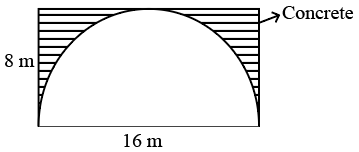Question 4

# Consider a cuboidal underground tunnel of length 500 m whose cross-section is given in the figure. If $$1 m^3$$ of concrete costs 1000 rupees, find the amount of money needed to build the Tunnel.Solution

Area of the tunnel to be filled with concrete = 16*8-$$\pi\ \left(32\right)$$

Length of the length along which the tunnel has to be filled with concrete = 500m

Volume = 16*8-$$\pi\ \left(32\right)$$*500

= $$32(4-\pi)$$*500

Cost of concrete per $$1 m^3$$ = 1000

Cost of $$32(4-\pi)$$*500 = $$32(4-\pi)$$*500*1000

=$$16(4-\pi)*10^{6}$$